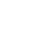# 关于Java正则表达式的基本用法和实例的相关资料介绍

Java正则表达式的基本用法和实例大全

1、英文句点.符号：匹配单个任意字符。

2、中括号[]：只有方括号里面指定的字符才参与匹配，也只能匹配单个字符。

3、| 符号。相当与“或”，可以匹配指定的字符，但是也只能选择其中一项进行匹配。

4、表示匹配次数的符号5、^符号：表示否，如果用在方括号内，^表示不想匹配的字符。

6、\S符号：非空字符

7、\s符号：空字符，只可以匹配一个空格、制表符、回车符、换页符，不可以匹配自己输入的多个空格。

8、\r符号：空格符，与\n、\tab相同

1、\d表示[0—9]

2、\D表示[^0—9]

3、\w表示[0—9A—Z_a—z]

4、\W表示[^0—9A—Z_a—z]

5、\s表示[\t\n\r\f]

6、\S表示[^\t\n\r\f]

1、Java：(([a-z]|_)(\\w*)){6,20}匹配以字母或下划线开头，字母数字下划线结尾的字符串

2、JavaScript：/^(\-?)(\d+)\$/匹配数字

3、JavaScript：/^\w+\$/匹配字母数字下划线。

public boolean matches(String regex)

```package Lemon;
import java.util.Scanner;
public class RegexDm {
public static void main(String[] args){
Scanner sc = new Scanner(System.in);
System.out.println("请输入手机好：");
String s = sc.nextLine();
String regex = "1\\d{9}";//定义手机好规则
boolean flag = s.matches(regex);//判断功能
System.out.println("flag:"+flag);
}
}
```

2、分割功能

public String[] split(String regex)

```package Lemon;
import java.util.Scanner;
public class RegexDm {
public static void main(String[] args){
String age = "18-24";//定义年龄范围
String regex = "-";
String[] strArr = age.split(regex);//分割成字符串数组
int startAge = Integer.parseInt(strArr);
int endAge = Integer.parseInt(strArr);
Scanner sc = new Scanner(System.in);
System.out.println("请输入您的年龄：");
int a = sc.nextInt();
if (a >= startAge && a <= endAge){
System.out.println("你就是我想找的");
}else{
System.out.println("滚");
}
}
}
```

3、替换功能

public String replaceAll(String regex,String replacement)

```package Lemon;
public class RegexDm {
public static void main(String[] args){
String s = "12342jasfkgnas234";
//把字符串里面的数字替换成*
String regex = "\\d";
String ss = "*";
String result = s.replaceAll(regex,ss);
System.out.println(result);
}
}
```

^\d+\$ ：非负整数（正整数 + 0）

^[0-9]*[1-9][0-9]*\$ ：正整数

^((-\d+)|(0+))\$ ：非正整数（负整数 + 0）

^-[0-9]*[1-9][0-9]*\$ ：负整数

^-?\d+\$ ：整数

^\d+(\.\d+)?\$ ：非负浮点数（正浮点数 + 0）

^(([0-9]+\.[0-9]*[1-9][0-9]*)|([0-9]*[1-9][0-9]*\.[0-9]+)|([0-9]*[1-9][0-9]*))\$ ：正浮点数

^((-\d+(\.\d+)?)|(0+(\.0+)?))\$ ：非正浮点数（负浮点数 + 0）

^(-(([0-9]+\.[0-9]*[1-9][0-9]*)|([0-9]*[1-9][0-9]*\.[0-9]+)|([0-9]*[1-9][0-9]*)))\$ ：负浮点数

^(-?\d+)(\.\d+)?\$ ：浮点数

^[A-Za-z]+\$ ：由26个英文字母组成的字符串

^[A-Z]+\$ ：由26个英文字母的大写组成的字符串

^[a-z]+\$ ：由26个英文字母的小写组成的字符串

^[A-Za-z0-9]+\$ ：由数字和26个英文字母组成的字符串

^\w+\$ ：由数字、26个英文字母或者下划线组成的字符串

^[\w-]+(\.[\w-]+)*@[\w-]+(\.[\w-]+)+\$ ：email地址

^[a-zA-z]+://(\w+(-\w+)*)(\.(\w+(-\w+)*))*(\?\S*)?\$ ：url

/^(d{2}|d{4})-((0([1-9]{1}))|(1[1|2]))-(([0-2]([1-9]{1}))|(3[0|1]))\$/ ：年-月-日

/^((0([1-9]{1}))|(1[1|2]))/(([0-2]([1-9]{1}))|(3[0|1]))/(d{2}|d{4})\$/ ：月/日/年

^([w-.]+)@(([[0-9]{1,3}.[0-9]{1,3}.[0-9]{1,3}.)|(([w-]+.)+))([a-zA-Z]{2,4}|[0-9]{1,3})(]?)\$ ：Emil

/^((\+?[0-9]{2,4}\-[0-9]{3,4}\-)|([0-9]{3,4}\-))?([0-9]{7,8})(\-[0-9]+)?\$/ ：电话号码

^(d{1,2}|1dd|2[0-4]d|25[0-5]).(d{1,2}|1dd|2[0-4]d|25[0-5]).(d{1,2}|1dd|2[0-4]d|25[0-5]).(d{1,2}|1dd|2[0-4]d|25[0-5])\$ ：IP地址

《关于Java正则表达式的基本用法和实例的相关资料介绍》